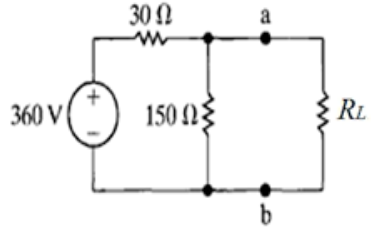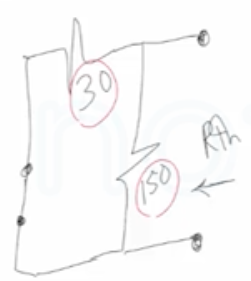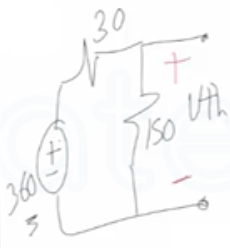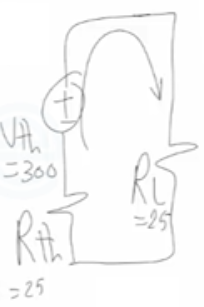Need Help?

Subscribe to Circuit

###### \${selected_topic_name}
• Notes

$\begin{array}{l}{\text { a) Find the value of } R_{L} \text { that results in maximum power being transferred to } R_{L} \text { . }} \\ {\text { b) Calculate the maximum power that can be delivered to } R_{L} .}\end{array}$(1)$R_{t h}=\frac{150 * 30}{150+30}=25 \Omega$

$R_{t h}=R_ L=25 \Omega$

(2)$V_{th}=\frac{150 * 360}{150+30}$

$V_{th}=300 V$$P_{\max }=\frac{V_{th}^2} {4R_{l}}$

$P_{max}=\frac{(300)^{2}}{4*25}$

$P_{max}=900w$